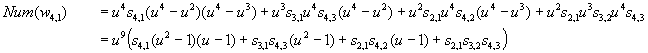Seeking coefficients of the superfunction of an analytic function

Preliminaries: Assume an analytic function defined by a power series without constant term:

f(x)=for plaintext-writing I use also  the easier notation f(x)=a x + b x2 + c x3+…

Use of matrices for functional composition: Composition and selfcomposition of analytic functions can be expressed elegantly using a matrix notation (also known as Bell-/Carleman-matrix). Consider the vectorial representation of f(x) as the dot-product of a vector V(x) = [1, x, x2, x3,…] containing the powers of x and another vector, A1 , containing the coefficients, such that

V(x)~ * A1 = 1*a0 + x*a1 + x2*a2 + …  = f(x)

The idea of composition/iteration of functions can then be expressed if we collect all vectors Ak into a matrix, where the Ak are defined that V(x)*Ak = f(x)k or said differently, that the generating function for Ak is f(x)k . Denote such a matrix A = [A0, A1, A2, A3,…] and we will have

V(x) ~ * A = [1, x, x2, x3,…] * A = [1, f(x), f(x)2, f(x)3,…] = V(f(x))~

Because the "input" and the "output" of that matrix-formula have the same type (a vector containing the consecutive powers of its parameter x, I call this a "vandermonde-vector") we can express iteration simply

V(x)~ * A = V(f(x))~
V(f(x))~ * A = V(f(f(x)) ~

and as long as the matrix-products A*A , A*A*A do not run into singularities we can even write

V(x)~ * Ah = V(f°h(x))~

where h is the h'th iterate of f.

For analytic functions having no constant term, as for instance exp(x)-1 , that matrices are lower triangular, the matrixpowers do not introduce singularities (each inner product has finitely many terms) and they even allow fractional powers and thus fractional iterates of the associated function.

 For compactness of notation I introduce the symbolic notation for a power divided by the agreeing factorial:Then the Bell-matrix A for a general analytic function f without constant term has infinite size, ist triangular and its top-left segment begins with(The top row includes a factorial which must be multiplied to each entry of the column.) Here the sum of the exponents agree to the column-index, and the sum of exponent*index of each product­term agrees with the row-index. It is just a complete partinioning scheme.

Example: the entry a3°1a2°2 is in column 3 because the sum of the exponents is 1+2=3, and it is in row 7 because exponents and indexes give 3*1 +2*2 = 7 . All combinations giving 3 and 7 this way are summands of that matrix-entry.

For easier reading written out in consecutive letters f(x)=ax + bx2 + cx3 + …Application to the exp-function: applied this to the exponential function requires
1! a = 2! b = 3! c = 4! d … = 1 and f(x) = exp(x)-1 .

Then we haveNote, that the resulting entries are just factorially scaled Stirlingnumbers 2nd kind.

We check one element:

A4,2 = (1/3! + (1/2!)²/2!)*2! = 1/3 + (1/2!)² = 7/12 = stirling2(4,2)*2!/4!

Further, we want this matrix to be the Bell-matrix for f(x)=tx –1 = exp(u*x) –1 for some base t other than e so we have to premultiply each row by a power of log(t) (which we call u):

At=The coefficients of the superfunction

The matrix of eigenvectors: What we're discussing in the MO-thread is the explicite description of the coefficients of the power series of the superfunction of exp(x) such that F(1+h) = exp(F(h))) . The expression for the linearizing function in Schröder's groundsetting article uses the infinite iterate as a limit to compute the coefficients of the powerseries for the "schröder-function". This is exactly the same functionality which is captured by the matrix of eigenvectors of a diagonalizable matrix: the matrix of eigenvectors can be seen as a scaled version of the infinite power of the diagonalizable matrix. Thus the diagonalization provides us with the coefficients for the powerseries of the Schröder-function for regular iteration.

We have to determine the eigenmatrix W such that

W-1 * D * W = At

and D is diagonal and contains the eigenvalues. For triangular matrices the eigenvalues are just the entries of the diagonal, so the the process of determining the eigenmatrix W (also normed to have diagonal 1) is very simple and straightforward. See the following Pari/GP-code:

{ EigenW(A) = local(W, dim); \\ diagonalization of a lower triangular matrix
dim = rows(A);
W = matid(dim); \\ note that in Pari/GP the row/col-indexes begin at 1
for(r = 2,dim,
forstep(c = r-1,1,-1,
W[r,c] = sum(k = 0,r-1-c, W[r,r-k] * A[r-k,c]) / (A[r,r] – A[c,c]) ))
return([W^-1,diag(A),W]); } \\ return all three matrices

Example: the computation of the top-left segment of W according to EigenW(A): (using one-level of recursion)

 1 0 1 0 1*A2,1 W2,1= ----------       A2,2-A1,1 1 0 1*A3,1+W3,2*A2,1 W3,1=-----------------         A3,3-A1,1 1*A3,2 W3,2=----------       A3,3-A2,2 1 0 1*A4,1+W4,3*A3,1+W4,2*A2,1 W4,1=---------------------------           A4,4-A1,1 1*A4,2+W4,3*A3,2 W4,2=-----------------           A4,4-A2,2 1*A4,3 W4,3=----------       A4,4-A3,3 1

Removing recursion: This definition contains recursion, but it is not deep and easily removeable.

Example entry W4,1: After expanding the recursive references W4,1 is explicitely:It may be helpful to make a common denominator and expand the resulting numerator to possibly see a more closed-form pattern.

Also we may introduce the current parameter u (the log of the base t here) and its powers into the formula:Now expand the remaining references Ar,c in the numerator. For the tx –1 – function these are just the factorially scaled Stirling numbers 2nd-kind and the appropriate power of log(t)  ( =u ):where sr,c = stirling2r,c*c!/r!

Even more explicite we could use the detailed decompositions of the Ar,c by factorials:Everything expanded and like powers of u collected:Here we see the four coefficients of your list in the fourth row: (6,6,5,1)

Gottfried Helms, 8.3.2011